### Example 3.1 Traveling Salesman Problem with Local Optimization

This example illustrates the use of the GA procedure to solve a traveling salesman problem (TSP); it combines a genetic algorithm with a local optimization strategy. The procedure finds the shortest tour of 20 locations randomly oriented on a two-dimensional-
plane, where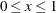and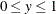. The location coordinates are input in the following DATA step:

/* 20 random locations for a  Traveling Salesman Problem */
data locations;
input x y;
datalines;
0.0333692 0.9925079
0.6020896 0.0168807
0.1532083 0.7020444
0.3181124 0.1469288
0.1878440 0.8679120
0.9786112 0.4925364
0.7918010 0.7943144
0.5145329 0.0363478
0.5500754 0.8324617
0.3893757 0.6635483
0.9641841 0.6400201
0.7718126 0.5463923
0.7549037 0.4584584
0.2837881 0.7733415
0.3308411 0.1974851
0.7977221 0.1193149
0.3221207 0.7930478
0.9201035 0.1186234
0.2397964 0.1448552
0.3967470 0.6716172
;


First, the GA procedure is run with no local optimizations applied:

proc ga data1 = locations seed = 5554;
call SetEncoding('S20');
ncities = 20;
array distances[20,20] /nosym;
do i = 1 to 20;
do j = 1 to i;
distances[i,j] = sqrt((x[i] - x[j])**2 + (y[i] - y[j])**2);
distances[j,i] = distances[i,j];
end;
end;
call SetObj('TSP',0,'distances', distances);
call SetCross('Order');
call SetMut('Invert');
call SetMutProb(0.05);
call SetCrossProb(0.8);
call SetElite(1);
call Initialize('DEFAULT',200);
call ContinueFor(140);
run;


The PROC GA statement uses a DATA1= option to get the contents of the locations data set, which creates array variablesandfrom the corresponding fields of the data set. A solution will be represented as a circular tour, modeled as a 20-element sequence of locations, which is set up with the SetEncoding call. The array variable distances is created, and the loop initializes distances from theandlocation coordinates such that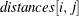is the Euclidean distance between locationand. Next, the SetObj call specifies that the GA procedure use the included TSP objective function with the distances array. Then, the genetic operators are specified, with SetCross for the crossover operator and SetMut for the mutation operator. Since the crossover probability and mutation probability are not explicitly set in this example, the default values of 1 and 0.05, respectively, are used. The selection parameters are not explicitly set (with SetSel and SetElite calls), so by default, a tournament of size 2 is used, and an elite parameter of 1 is used. Next, the Initialize call specifies default initialization (random sequences) and a population size of 100. The ContinueFor call specifies a run of 220 iterations. This value is a result of experimentation, after it was determined that the solution did not improve with more iterations. The output of this run of PROC GA is given in Output 3.1.1.

Output 3.1.1: Simple Traveling Salesman Problem

PROC GA Optimum Values

Objective
3.7465311323

Solution
Element Value
1 12
2 13
3 18
4 16
5 2
6 8
7 15
8 4
9 19
10 3
11 1
12 5
13 14
14 17
15 10
16 20
17 9
18 7
19 11
20 6

The following program illustrates how the problem can be solved in fewer iterations by employing a local optimization. Inside the user objective function, before computing the objective value, every adjacent pair of cities in the tour is checked to determine if reversing the pair order would improve the objective value. For a pair of locations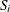and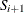, this means comparing the distance traversed by the subsequenceto the distance traversed by the subsequence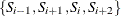, with appropriate wrap-around at the endpoints of the sequence. If the distance for the swapped pair is smaller than the original pair, then the reversal is done, and the improved solution is written back to the population.

proc ga data1 = locations seed = 5554;
call SetEncoding('S20');
ncities = 20;
array distances[20,20] /nosym;
do i = 1 to 20;
do j = 1 to i;
distances[i,j] = sqrt((x[i] - x[j])**2 + (y[i] - y[j])**2);
distances[j,i] = distances[i,j];
end;
end;
/* Objective function with local optimization */
function TSPSwap(selected[*],ncities,distances[*,*]);
array s /nosym;
call dynamic_array(s,ncities);
/* First try to improve solution by swapping adjacent cities */
do i = 1 to ncities;
city1 = s[i];
inext = 1 + mod(i,ncities);
city2 = s[inext];
if i=1 then
before = s[ncities];
else
before = s[i-1];
after = s[1 + mod(inext,ncities)];
if (distances[before,city1]+distances[city2,after]) >
(distances[before,city2]+distances[city1,after]) then do;
s[i] = city2;
s[inext] = city1;
end;
end;
call WriteMember(selected,1,s);
/* Now compute distance of tour */
distance = distances[s[ncities],s];
do i = 1 to (ncities - 1);
distance + distances[s[i],s[i+1]];
end;
return(distance);
endsub;
call SetObjFunc('TSPSwap',0);
call SetCross('Order');
call SetMut('Invert');
call SetMutProb(0.05);
call SetCrossProb(0.8);
call SetElite(1);
call Initialize('DEFAULT',200);
call ContinueFor(35);
run;


The output after 85 iterations is given in Output 3.1.2.

Output 3.1.2: Traveling Salesman Problem with Local Optimization

PROC GA Optimum Values

Objective
3.7465311323

Solution
Element Value
1 13
2 18
3 16
4 2
5 8
6 15
7 4
8 19
9 3
10 1
11 5
12 14
13 17
14 10
15 20
16 9
17 7
18 11
19 6
20 12

Since all tours are circular, the actual starting point does not matter, and this solution is equivalent to that reached with the simple approach without local optimization. It is reached after only 85 iterations, versus 220 with the simple approach.

Note: See the Examples section in Chapter 2: The OPTNET Procedure in SAS/OR 12.1 User's Guide: Network Optimization Algorithms . for an example of how to use PROC OPTNET to solve the TSP.